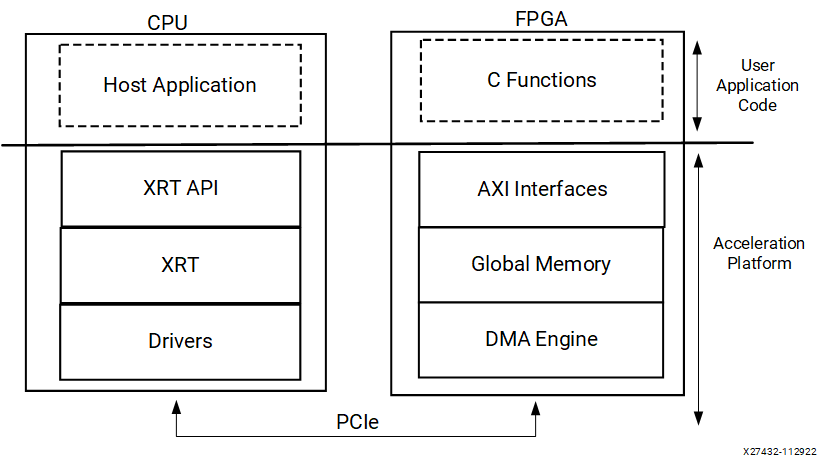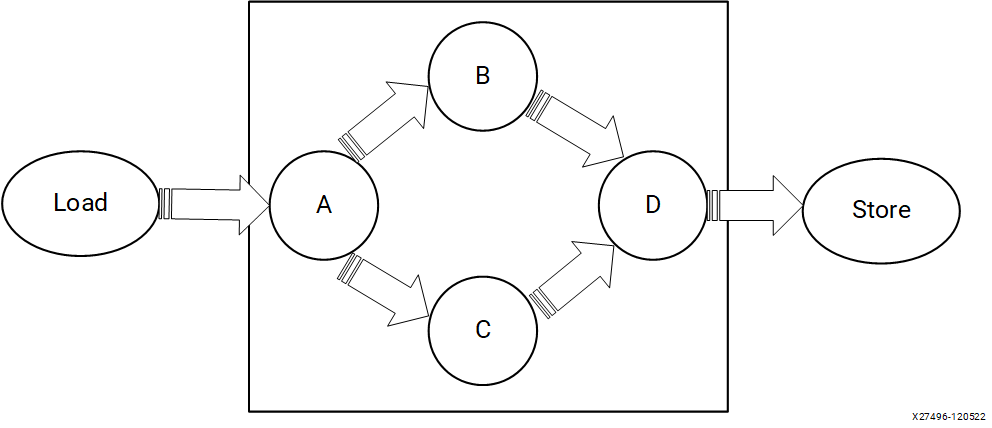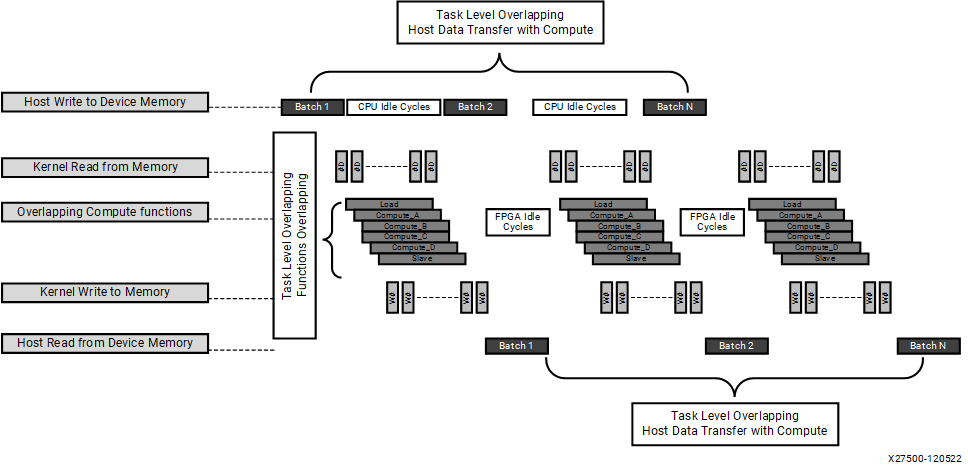# A Sample Application - 2022.2 English

## Vitis Unified Software Platform Documentation: Application Acceleration Development (UG1393)

Document ID
UG1393
Release Date
2022-12-07
Version
2022.2 English

This section provides a snapshot of the evolution of a program written for CPU into an application written for FPGA-based acceleration. This section is primarily intended to showcase key ideas for building your application without going into details. You may come across several new terms here, but you can refer to Terminology for some definitions.

The figure below illustrates the execution flow of the Vitis application acceleration environment. The application program is split between an application running on CPU (called the host program) and hardware-accelerated kernels running on FPGA with a communication channel between them. The host program, written in C/C++ and using the XRT API, is compiled into an executable that runs on an x86 based host processor while hardware-accelerated kernels are compiled into an executable device binary (.xclbin) that runs within the programmable logic (PL) region of an Xilinx device on the Alveo accelerator card.

Figure 1. CPU/FPGA InteractionThe API calls managed by XRT are used to process transactions between the host program and the hardware accelerators. Communication between the host and the kernel, including control and data transfers, occurs across the PCIe bus. The execution model of a Vitis application can be broken down into the following steps:

1. The host program writes the data needed by a kernel into the global memory of the attached device through the PCIe interface on an Alveo Data Center accelerator card.
2. The host program sets up the kernel with its input parameters.
3. The host program triggers the execution of the kernel function on the FPGA.
4. The kernel performs the required computation while reading data from global memory, as necessary.
5. The kernel writes data back to global memory and notifies the host that it has completed its task.
6. The host program reads data back from global memory into the host memory and continues processing as needed.

The following is a simple program written in C++ for execution on the CPU. This program includes the `compute()` function to be accelerated as a kernel on an Alveo accelerator card.

``````#include <vector>
#include <iostream>
#include <ap_int.h>
#include "hls_vector.h"

#define  totalNumWords 512
unsigned char data_t;

int main(int, char**) {
// initialize input vector arrays on CPU
for (int i = 0; i < totalNumWords; i++) {
in[i] = i;
}
compute(data_t in[totalNumWords], data_t Out[totalNumWords]);
check_results();
}

void compute (data_t in[totalNumWords ], data_t Out[totalNumWords ]) {
data_t tmp1[totalNumWords], tmp2[totalNumWords];
A: for (int i = 0; i < totalNumWords ; ++i) {
tmp1[i] = in[i] * 3;
tmp2[i] = in[i] * 3;
}
B: for (int i = 0; i < totalNumWords ; ++i) {
tmp1[i] = tmp1[i] + 25;
}
C: for (int i = 0; i < totalNumWords ; ++i) {
tmp2[i] = tmp2[i] * 2;
}
D: for (int i = 0; i <  totalNumWords ; ++i) {
out[i] = tmp1[i] + tmp2[i] * 2;
}
}``````

The program looks very similar to any other C++ program where there the main function calls a compute function, setting up the data to be sent to compute function, and checking the results with golden results after compute function completes. The execution of this program is sequential on the CPU. This program can also run sequentially on an FPGA, producing correct results without any performance gain compared to the CPU. For the application to execute with higher performance on an FPGA, the program needs to be re-architected to enable parallelism at various levels. Examples of parallelism can include:

• The compute function can start before all the data is transferred from the host to the compute function
• Multiple compute functions can run in an overlapping fashion, for example a "for" loop can start the next iteration before the previous iteration has completed
• The operations within a "for" loop can run concurrently on multiple words and doesn't need to be executed on a per-word basis

You will need to re-architect the compute function that resides on the FPGA as an accelerated kernel, and the host application that runs on the CPU and communicates with the accelerated kernels.

## Re-Architecting Kernel Code

From the prior example it is the `compute()` function that needs to be re-architected for FPGA-based acceleration.

In the `compute()` function Loop A multiplies the input with 3 and creates two separate paths, B and C. Loop B and C performs operations and feed the data to D. This is a simple representation of a realistic case where you have several tasks to be performed one after another and these tasks are connected to each other as a network like the one shown below.

Figure 2. Kernel ArchitectureHere are the key takeaways for re-architecting the kernel code are:

• Task-level parallelism is implemented at the function level. To implement task-level parallelism loops are pushed into separate functions. The original `compute()` function is split into multiple sub-functions. As a rule of thumb, sequential functions can be made to execute concurrently, but sequential loops will execute sequentially.
• These tasks (or sub-functions) are communicating with each other using `hls::stream` which acts as a FIFO channel. The `hls::stream` class is a C++ template class for modeling streams behavior between functions.
• Instruction-level parallelism is implemented by reading 16 32-bit words from memory (or 512-bits of data). Computations can be performed on all these words in parallel. The `hls::vector` class is a C++ template class for executing vector operations on multiple samples concurrently.
• The `compute()` function needs to be re-architected into load-compute-store sub-functions, as shown in the example below. The load and store functions encapsulate the data accesses and isolate the computations performed by the various compute functions.
• Additionally, there are compiler directives starting with `#pragma` that can transform the sequential code into parallel execution.
``````#include "diamond.h"
#define NUM_WORDS 16
extern "C" {

void diamond(vecOf16Words* vecIn, vecOf16Words* vecOut, int size)
{
hls::stream<vecOf16Words> c0, c1, c2, c3, c4, c5;
assert(size % 16 == 0);

#pragma HLS dataflow
compute_A(c0, c1, c2, size);
compute_B(c1, c3, size);
compute_C(c2, c4, size);
compute_D(c3, c4,c5, size);
store(c5, vecOut, size);
}
}

void load(vecOf16Words *in, hls::stream<vecOf16Words >& out, int size)
{
Loop0:
for (int i = 0; i < size; i++)
{
#pragma HLS performance target_ti=32
#pragma HLS LOOP_TRIPCOUNT max=32
out.write(in[i]);
}
}

void compute_A(hls::stream<vecOf16Words >& in, hls::stream<vecOf16Words >& out1, hls::stream<vecOf16Words >& out2, int size)
{
Loop0:
for (int i = 0; i < size; i++)
{
#pragma HLS performance target_ti=32
#pragma HLS LOOP_TRIPCOUNT max=32
out1.write(t * 3);
out2.write(t * 3);
}
}
void compute_B(hls::stream<vecOf16Words >& in, hls::stream<vecOf16Words >& out, int size)
{
Loop0:
for (int i = 0; i < size; i++)
{
#pragma HLS performance target_ti=32
#pragma HLS LOOP_TRIPCOUNT max=32
}
}

void compute_C(hls::stream<vecOf16Words >& in, hls::stream<vecOf16Words >& out, int size)
{
Loop0:
for (data_t i = 0; i < size; i++)
{
#pragma HLS performance target_ti=32
#pragma HLS LOOP_TRIPCOUNT max=32
}
}
void compute_D(hls::stream<vecOf16Words >& in1, hls::stream<vecOf16Words >& in2, hls::stream<vecOf16Words >& out, int size)
{
Loop0:
for (data_t i = 0; i < size; i++)
{
#pragma HLS performance target_ti=32
#pragma HLS LOOP_TRIPCOUNT max=32
}
}

void store(hls::stream<vecOf16Words >& in, vecOf16Words *out, int size)
{
Loop0:
for (int i = 0; i < size; i++)
{
#pragma HLS performance target_ti=32
#pragma HLS LOOP_TRIPCOUNT max=32
}
}``````

## Re-Architecting the Host Application

The main function in the original program is responsible for setting up the data, calling the compute function, checking the results, etc. In the case of an accelerated application, the host code is responsible for initializing the data to be sent/received over the PCIe® bus to the device memory. It also sets the kernel function arguments similar to how the main function calls compute functions. The API calls, managed by XRT, are used to process transactions between the host program and the hardware accelerators.

In general, the structure of the host application can be divided into the following steps:

2. Allocate buffers in the global memory
3. Create the input test data and map the buffers to the host memory
4. Setting up the kernel and kernel arguments.
5. Transferring buffers between the host and kernels
6. Execute the kernel.
7. Receive the output results back to the host into output buffers

The host application re-written for the `compute()` function described above, making use of the XRT native API to run on the Alveo accelerator card is shown below:

``````// XRT includes
#include "experimental/xrt_bo.h"
#include "experimental/xrt_device.h"
#include "experimental/xrt_kernel.h"

#include "types.h"

int main(int argc, char** argv) {
unsigned int device_index = 0;

size_t vector_size_bytes = sizeof(int) * totalNumWords;
auto krnl = xrt::kernel(device, uuid, "diamond");

std::cout << "Allocate Buffer in Global Memory\n";
auto bufIn = xrt::bo(device, vector_size_bytes, krnl.group_id(0));
auto bufOut = xrt::bo(device, vector_size_bytes, krnl.group_id(1));

// Map the contents of the buffer object into host memory
auto bufIn_map = bufIn.map<int*>();
auto bufOut_map = bufOut.map<int*>();
std::fill(bufIn_map, bufIn_map + totalNumWords, 0);
std::fill(bufOut_map, bufOut_map + totalNumWords, 0);

// Create the input data
for (int i = 0; i < totalNumWords; i++)
bufIn_map[i] = (uint32_t)i;

// Create the output golden data
int bufReference[totalNumWords];
for (int i = 0; i < totalNumWords; ++i) {
bufReference[i] = ((i*3)+25)+((i*3)*2);
}

// Synchronize buffer content with device side
bufIn.sync(XCL_BO_SYNC_BO_TO_DEVICE);

std::cout << "Execution of the kernel\n";
auto run = krnl(bufIn,bufOut,totalNumWords/16);
run.wait();

// Get the output;
std::cout << "Get the output data from the device" << std::endl;
bufOut.sync(XCL_BO_SYNC_BO_FROM_DEVICE);

for (int i = 0; i < totalNumWords; i++)
{
std::cout << "Referece  "  << bufReference[i] << std::endl;
std::cout << "Out  "  << bufOut_map[i] << std::endl;
}

// Validate our results
if (std::memcmp(bufOut_map, bufReference, totalNumWords))
throw std::runtime_error("Value read back does not match reference");
std::cout << "TEST PASSED\n";
``````

## Application Execution Timeline

When run on the Alveo accelerator card, the application timeline look like the following.

Figure 3. Application TimelineThe execution of the application on an FPGA is quite different than on a CPU due to several types of parallelism that can be observed from figure above. The kernel code was written to leverage task-level parallelism by creating sub-functions for each loop. The result is that `compute_A`, `compute_B`, `compute_C`, and `compute_D` are running in an overlapping fashion. In fact, `compute_A`, `compute_B`, `compute_C`, and `compute_D` are sub-function calls within the compute function. A similar execution overlap can be accomplished for multiple kernels.

While the hardware device and its kernels are designed to offer potential parallelism, the software application must be engineered to take advantage of this potential parallelism. Task-level parallelism is further enabled by overlapping host-to-device data transfers as well as overlapping the compute function execution.